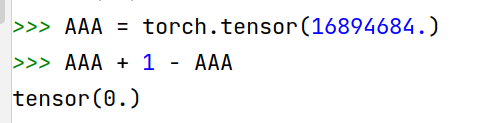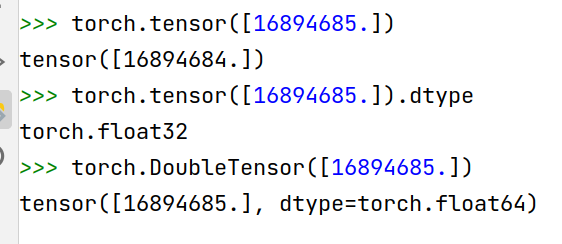# The number of '16894685.' can not compute by PyTorch.

I found a very strange phenomenon. Specifically, I initialized a data AAA = torch.tensor(16894685.). However, when I try to print this value, the number displayed is ‘16894684.’. Then I tried to make it print 1. using AAA + 1 - AAA, but the weird thing happened again. The result of the calculation is 0, which makes my experiments impossible to continue, because the loss will be NAN. When I convert tensor to numpy type data, the calculation result is normal. I’m very confused and looking forward to someone who can help me with my doubts. The pictures show the result of my program running.This is expected as you are using `float32` as the default `dtype` in PyTorch, which starts to round to multiple of 2 (even numbers) in the interval of `[2**24, 2**25]`, which your value is in. Take a look at this Wikipedia page for more information and check the `Precision limitations on integer values` section.

`numpy` uses `float64` by default and transforming your PyTorch tensor to `float64` too would work:

``````AAA = torch.tensor(16894685., dtype=torch.float64)
AAA + 1 - AAA
``````

Thank you very much. I looked at the float type as you suggested and it does exactly what you said. When I use float 64, the above problem is solved fine. Thank you again!